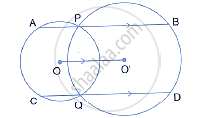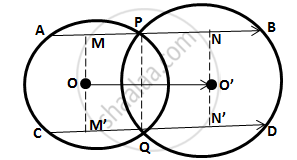Share

# In the Following Figure; P and Q Are the Points of Intersection of Two Circles with Centres O and O’. If Straight Lines Apb and Cqd Are Parallel to O O'; Prove That: (I) O O' = 1/2ab (Ii) Ab = Cd - Mathematics

Course

#### Question

In the following figure; P and Q are the points of intersection of two circles with centres O and O’. If straight lines APB and CQD are parallel to O O'; prove that:

(i) O O' = 1/2AB (ii) AB = CD#### Solution

Drop OM and O'N perpendicular on AB and OM' and O'N' perpendicular on CD.∴ MP = 1/2AP,PN=1/2 BP, M'Q =1/2CQ, QN'=1/2QD

Now, OO ' = MN = MP + PN = 1/2 (AP + BP) = 1/2 AB         ---------(i)

And OO ' = M ' N ' = M 'Q + QN ' = 1/2 (CQ + QD) = 1/2 CD           -------(ii)
By (i) and (ii)
AB = CD

Is there an error in this question or solution?
In the Following Figure; P and Q Are the Points of Intersection of Two Circles with Centres O and O’. If Straight Lines Apb and Cqd Are Parallel to O O'; Prove That: (I) O O' = 1/2ab (Ii) Ab = Cd Concept: Chord Properties - a Straight Line Drawn from the Center of a Circle to Bisect a Chord Which is Not a Diameter is at Right Angles to the Chord.